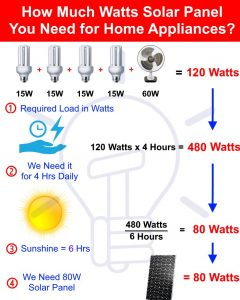Phone No

+92-321-8209843 | +92-323-2881289EmailGet A Quote

# ELECTRICAL TECHNOLOGY

## How to Select the Proper Solar Panel Rating for Household Appliances?

If you are going to install a solar panel system (off grid or on grid) and want to find the exact amount of wattage and solar panel rating, you can do it easily by the following simple method. The solved example and explanation are as follow.

Suppose we want to power up four lights each of 15 watts and a fan of 60 watts and we need to use these 4 lights and 1 fan for 4 hours every day. So first, we will calculate total watts usage.

PTotal= (4 x 15W) + 60W = 120 Watts.

This is our daily load per hour in watts we need to power up by solar panels.

We Need it for 4 Hrs Daily

Now, we need a continuous power supply for 4 hours a day by solar panel to the load.

Therefore, multiply 120 Watts with 4 hours.

PDaily = 120 W x 4 Hrs = 480 Watt Hours per day.

Sunshine = 6 Hrs

We are going to use 480 Watts daily for 4 hours. Let’s say we are having a complete sunshine for 6 hours each day.

Now we divide 480W by 6 hours to get the final rating of solar panel in watts. This way, we will get hourly power charge that we need for electrical appliances.

Rating of Solar Panel

PHourly = 480 W / 6 Hrs = 80 W / H

So you need a 80 watt solar panel.

Its mean, you need 480 watts for 4 hours where 80W solar panel will produce 480 Watts as sunshine is 6 hours. To know the battery bank, inverter and charge controller size for this system, see the link in the foot-note.Key Point:

The above calculations are based on Ideal case. Therefore, it is recommended that always choose a panel some bigger in rating than we need. Because when solar panel charges the battery, there is wastage of power due to losses as well.